Please listen to this song for inspiration during this homework.

## Exercise 1

Suppose you are a Pokémon Trainer in the Kanto region. You are currently walking through the tall grass of Viridian Forest on your way to Viridian City. When walking through talk grass, you will encounter wild Pokémon. Pokémon encounters happen one-at-a-time and you may assume they are independent.

In Viridian Forest, there are five potential Pokémon that you may encounter. The following shows the probability that any one encounter is a particular Pokémon, as well as the Pokémon’s type.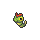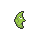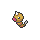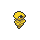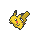Pokémon Caterpie Metapod Weedle Kakuna Pikachu
Probability 0.05 0.05 0.50 0.35 0.05
Type Bug Bug Bug, Poison Bug, Poison Electric

(a) Suppose you encounter 10 Pokémon. What is the probability that at least 1 is a Pikachu?

Solution:

Define $$X$$ as “number of Pikachus in 10 Pokémon.” Then, $$X$$ has a binomial distribution.

$X \sim b(n = 10, p = 0.05)$

\begin{aligned} P[X \geq 1] &= 1 - P[X = 0] \\ &= 1 - {{10}\choose{0}}(0.05)^{0}(0.95)^{10} \\ &= 1 - (0.95)^{10} \approx \boxed{0.40126} \end{aligned}

(b) Suppose you encounter 10 Pokémon. What is the probability that more than 7 are type poison?

Solution:

Define $$Y$$ as “number of Poisson Pokémon in 10 Pokémon.” Then, $$Y$$ has a binomial distribution.

$Y \sim b(n = 10, p = 0.85)$

\begin{aligned} P[X > 7] &= f(8) + f(9) + f(10) \\ &= {{10}\choose{8}}(0.85)^{8}(0.15)^{2} + {{10}\choose{9}}(0.85)^{9}(0.15)^{1} + {{10}\choose{10}}(0.85)^{10}(0.15)^{0} \\ &= 0.2758967 + 0.3474254 + 0.1968744 \approx \boxed{0.8201965} \end{aligned}

(c) What is the probability that you see your first Pikachu after your tenth encounter?

Solution:

Define $$Z$$ as “encounters until first Pikachu.” Then, $$Z$$ has a geometric distribution.

$Z \sim Geom(p = 0.05)$

$P[Z > 10] = (1 - 0.05)^{10} \approx \boxed{0.598737}$

(d) What is the probability that you see your third Pikachu on your twelfth encounter?

Solution:

Define $$W$$ as “encounters until third Pikachu.” Then, $$W$$ has a negative binomial distribution.

$W \sim NB(k = 3, p = 0.05)$

$P[W = 12] = {{11}\choose{2}}(0.05)^{3}(0.95)^{9} \approx \boxed{0.004333}$

(e) Suppose you encounter 8 Pokémon. What is the probability that it is 2 Pikachus, 4 Weedles and 2 Kakunas?

Solution:

$\frac{8!}{0!0!4!2!2!}(0.05)^{0}(0.05)^{0}(0.50)^{4}(0.35)^{2}(0.05)^{2} \approx \boxed{0.008039062}$

## Exercise 2

The Pokémon probabilities in Exercise 1 are from the 1998 video game Pokémon Red. Also released at the same time was Pokémon Blue. The games are extremely similar. One difference, is the proportion of certain Pokémon found in certain locations. For example, in Viridian Forest, the proportion of Metapod is:

• Pokémon Red: 0.05
• Pokémon Blue: 0.35